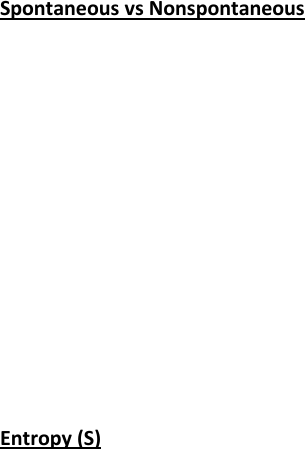Textbook Notes (290,000)
CA (170,000)
UofM (1,000)
CHEM (50)
Chapter

# CHEM 1310 Chapter Notes -Thermodynamics, Boiling Point, Standard Molar Entropy

Page:
of 2Spontaneous vs Nonspontaneous
• Spontaneous
Process or action that continues to
occur without external stimulus once
the action has been started
Reactions do not require constant
input to occur
• Non-spontaneous
Process or action that requires
constant external stimulus to
continue
Reactions require constant input to
occur
• If a process is spontaneous then
the reverse process is nonspontaneous
Entropy (S)
• Is a state function with specified
temperature, pressure and compositions
• Measure of energy at a microscopic
level
Particles may enter into energy levels/states
different ways based upon the amount of
energy in the system
The more energy, the more states partially
filled and the larger the amount of entropy
Based on Boltzmann’s equation (S = k ln W)
and particles-in-a-box
• In general:
Increased T increased entropy
Increased # gas molecules by reaction
increased entropy
Increased disorder/motions increased
entropy
(Practice) Example:
Entropy (S)
• Calculation of entropy
For a reversible reaction:
• A reaction that may change direction with
a very, very small change in some property
For a phase transition:
• Normal boiling point of a non-hydrogen
bonded organic liquid is usually around 87
JK-1mol-1
To relate standard to non-standard:
• Use a variation of the ideal gas law and
Raoult’s law to relate
S = Sᵒ - RlnP
S = Sᵒ - RlnC
(Practice) Examples:
Entropy (S)
• Second Law of Thermodynamics:
All spontaneous processes/actions
cause an increase in the entropy of
the universe
ΔStotal=ΔSuniverse=ΔSsystem+ΔSsurroundings> 0
An increase in entropy spontaneous
reaction
A decrease in entropy non-spontaneous
reaction
When entropy is zero reaction is at
equilibrium
Need an increase in the entropy of the
system or the surroundings (or both) for a
reaction to occur spontaneously
(Practice) Example: Section Exercises:
Entropy (S)
• Third Law of Thermodynamics:
The entropy of a pure perfect crystal at
0K is zero
Allows a reference point for the calculation of
standard molar entropies (So, Appendix D)
In general, more complex molecules
increased So
For the change in standard molar entropy of a
reaction:
=Σ (products) −Σ (reactants)